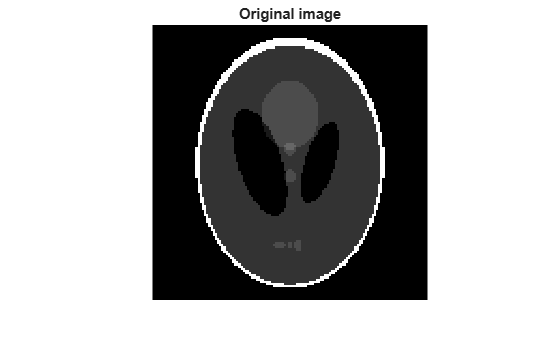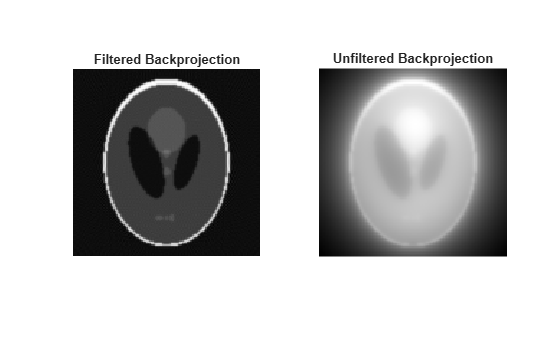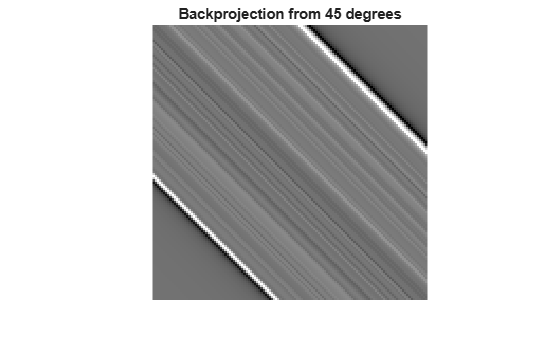Documentation

## Syntax

``I = iradon(R,theta)``
``I = iradon(R,theta,interp,filter,frequency_scaling,output_size)``
``[I,H] = iradon(___)``

## Description

example

````I = iradon(R,theta)` reconstructs the image `I` from projection data in `R`. `theta` describes the angles (in degrees) at which the projections were taken.You optionally can compute the inverse Radon transform using a GPU (requires Parallel Computing Toolbox™).```
````I = iradon(R,theta,interp,filter,frequency_scaling,output_size)` specifies parameters to use in the inverse Radon transform. You can specify any combination of the last four arguments. `iradon` uses default values for arguments that you omit.```
````[I,H] = iradon(___)` also returns the frequency response of the filter, `H`.```

## Examples

collapse all

Create an image of the phantom. Display the image.

```P = phantom(128); imshow(P) title('Original image')```Perform a Radon transform of the image.

`R = radon(P,0:179);`

Perform filtered backprojection.

`I1 = iradon(R,0:179);`

Perform unfiltered backprojection.

`I2 = iradon(R,0:179,'linear','none');`

Display the reconstructed images.

```figure subplot(1,2,1) imshow(I1,[]) title('Filtered Backprojection') subplot(1,2,2) imshow(I2,[]) title('Unfiltered Backprojection')```Create an image of the phantom.

`P = phantom(128);`

Perform a Radon transform of the image, then get the projection vector corresponding to a projection at a 45 degree angle.

```R = radon(P,0:179); r45 = R(:,46);```

Perform the inverse Radon transform of this single projection vector. The `iradon` syntax does not allow you to do this directly, because if `theta` is a scalar it is treated as an increment. You can accomplish the task by passing in two copies of the projection vector and then dividing the result by 2.

`I = iradon([r45 r45], [45 45])/2;`

Display the result.

```imshow(I, []) title('Backprojection from 45 degrees')```## Input Arguments

collapse all

Parallel beam projection data, specified as one of the following.

• If `theta` is a scalar, then specify `R` as a numeric column vector containing the Radon transform for `theta` degrees.

• If `theta` is a vector, then specify `R` as a 2-D matrix in which each column is the Radon transform for one of the angles in `theta`.

Data Types: `single` | `double` | `int8` | `int16` | `int32` | `int64` | `uint8` | `uint16` | `uint32` | `uint64` | `logical`

Projection angles (in degrees), specified as one of the following.

ValueDescription
numeric vectorProjection angles. There must be equal spacing between the angles.
numeric scalarIncremental angle between projections. Projections are taken at angles `m*theta`, where ```m = 0,1,2,...,size(R,2)-1```.
`[]`Automatically set the incremental angle between projections to `180/size(R,2)`

Data Types: `double`

Type of interpolation to use in the back projection, specified as one of these values, listed in order of increasing accuracy and computational complexity.

Value

Description

`'nearest'`

Nearest-neighbor interpolation

`'linear'`

Linear interpolation (the default)

`'spline'`

Spline interpolation

`'pchip'`Shape-preserving piecewise cubic interpolation

Data Types: `char` | `string`

Filter to use for frequency domain filtering, specified as one of these values.

Value

Description

`'Ram-Lak'`

Cropped Ram-Lak or ramp filter. The frequency response of this filter is | `f` |. Because this filter is sensitive to noise in the projections, one of the filters listed below might be preferable. These filters multiply the Ram-Lak filter by a window that de-emphasizes high frequencies.

`'Shepp-Logan'`

Multiplies the Ram-Lak filter by a `sinc` function

`'Cosine'`

Multiplies the Ram-Lak filter by a `cosine` function

`'Hamming'`

Multiplies the Ram-Lak filter by a Hamming window

`'Hann'`

Multiplies the Ram-Lak filter by a Hann window

`'None'`No filtering. `iradon` returns unfiltered backprojection data.

Data Types: `char` | `string`

Scale factor for rescaling the frequency axis, specified as a positive number in the range (0, 1]. If `frequency_scaling` is less than 1, then the filter is compressed to fit into the frequency range `[0,frequency_scaling]`, in normalized frequencies; all frequencies above ` frequency_scaling` are set to `0`.

Number of rows and columns in the reconstructed image, specified as a positive integer. If `output_size` is not specified, the size is determined from the length of the projections according to:

`output_size = 2*floor(size(`R`,1)/(2*sqrt(2)))`

If you specify `output_size`, then `iradon` reconstructs a smaller or larger portion of the image but does not change the scaling of the data. If the projections were calculated with the `radon` function, then the reconstructed image might not be the same size as the original image.

## Output Arguments

collapse all

Grayscale image, returned as a numeric matrix. If input projection data `R` is data type `single`, then `I` is `single`; otherwise `I` is `double`.

Data Types: `single` | `double`

Frequency response of the filter, returned as a numeric vector.

Data Types: `double`

## Algorithms

`iradon` assumes that the center of rotation is the center point of the projections, which is defined as `ceil(size(R,1)/2)`.

`iradon` uses the filtered back projection algorithm to perform the inverse Radon transform. The filter is designed directly in the frequency domain and then multiplied by the FFT of the projections. The projections are zero-padded to a power of 2 before filtering to prevent spatial domain aliasing and to speed up the FFT.

 Kak, A. C., and M. Slaney, Principles of Computerized Tomographic Imaging, New York, NY, IEEE Press, 1988.